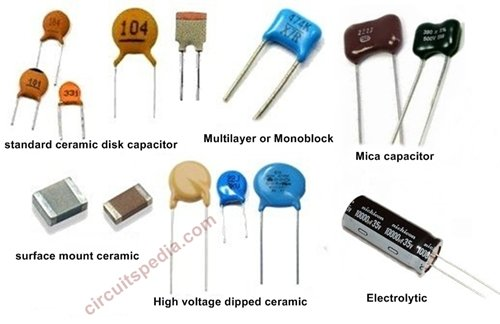Very Simple Method to Read Value of Capacitor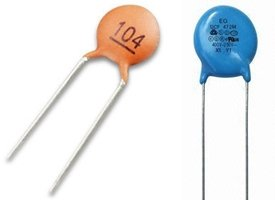Method of Finding the value/Meaning of codes of capacitor

Click Here For Resistor Colour Code And SMD Resistor Code

•  Ceramic disc capacitors have two to three digits code  printed on them.

•  The first two numbers describe the value of the capacitor and the third number is the number of zeros in the multiplier.

•   When the first two numbers are multiplied with the multiplier, the resulting value is the value of the capacitor in picofarads.

•  If there is only two number,  it means there is no multiplier, Then you just read the value of the first two numbers in picofarads.

•  If any capacitor has 10 printed on it- Then its value is 10 PF

•  When Any capacitor has 104 printed- It has multiplier of 4 (Third number of code ). 10 is multiplied by 10×104 =10000.  Then its value is 10×10000= 100000PF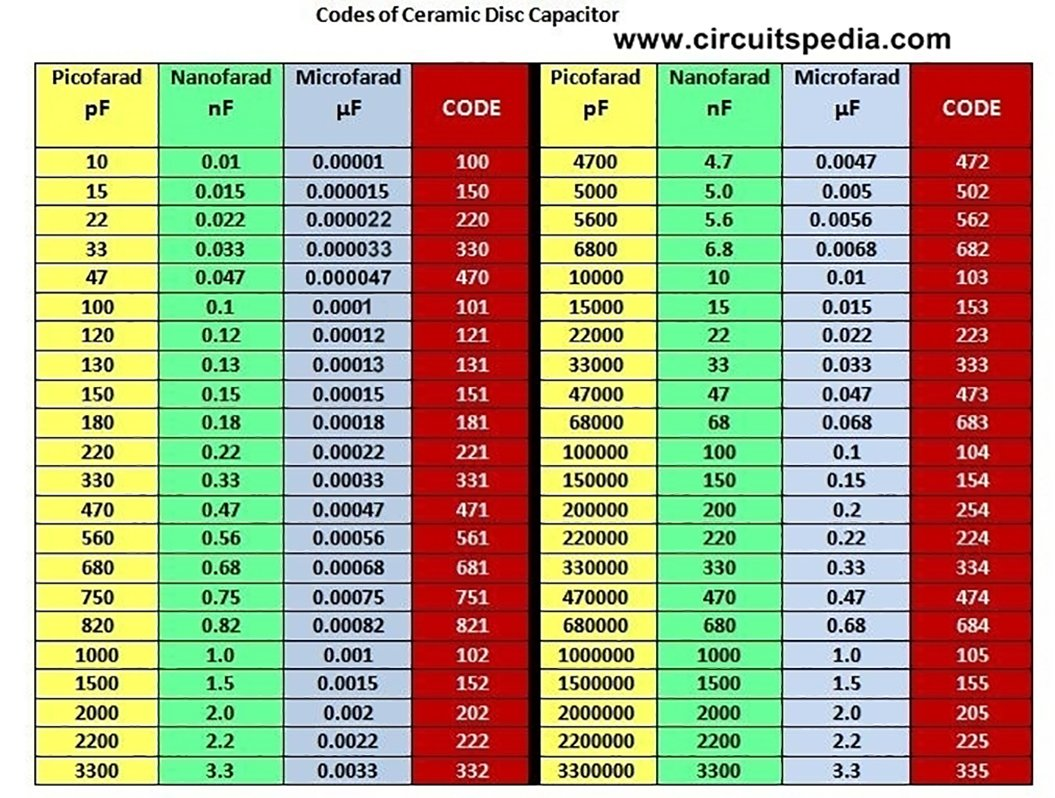Last number is the power of 10, and multiply with first two no.

If a capacitor has 682 code – first check the last no , here last no is 2. Now  the multiplier is 102

For example---

• 204= 20×104 =200000 PF
• 472=47×102 = 4700 PF
• 502=52×102 =5200 PF
• 330=33×100 =33 PF   [100 = 1]

Here is a Table of Mostly Used Codes of Ceramic Capacitor and their unit conversion in Micro,Nano and Picofarad

UNITS---

• Nano= 10-9
• Micro= 10-6

1 nF = 1000 pF
1 pF = 0.001 nF

Example:

convert 15 nF to pF:
15 nF = 15 × 1000 pF = 15000 pF

Codes of Polyester Film and Metallized Film Capacitor

If a capacitor is marked  with 2A474J, the capacitance is decoded as described above, the two first signs is the voltage rating and can be decoded from table given below here . 2A is 100VDC rating according to the EIA (Electronic industries alliance) standard.

A second letter will be a temperature coefficient if its present.

Some capacitors are only marked as 0.1 or 0.01, mostly in these cases the values are given in uF.

Some small capacitance capacitors can be marked with a R between numbers. If code is 3R9 then R is a indicator of values Less than 10pF and have nothing to do with resistance. 3R9 would be 3.9pF.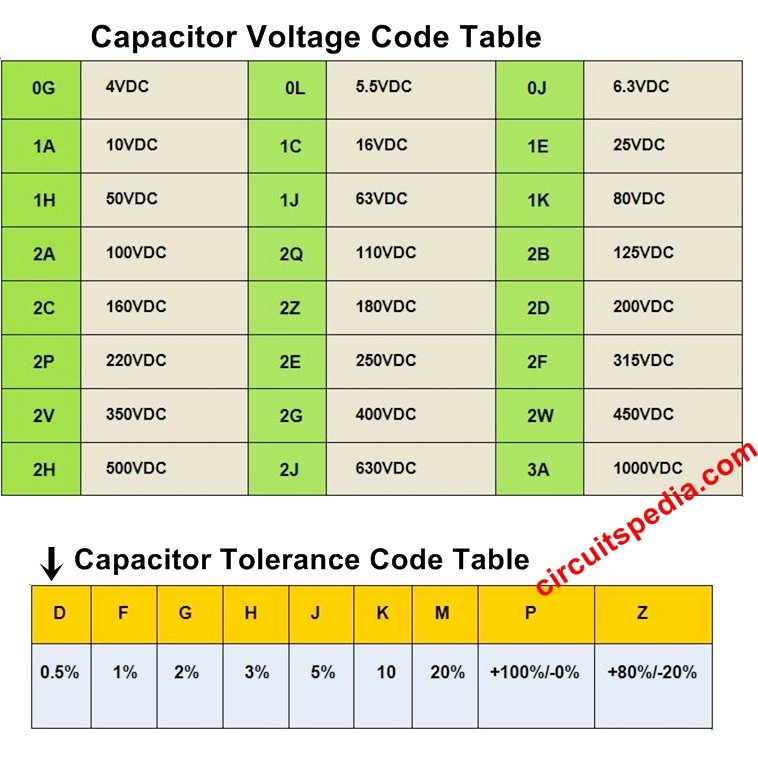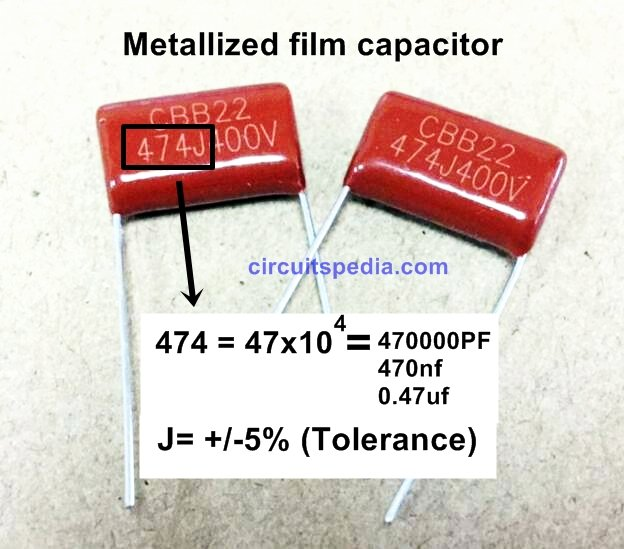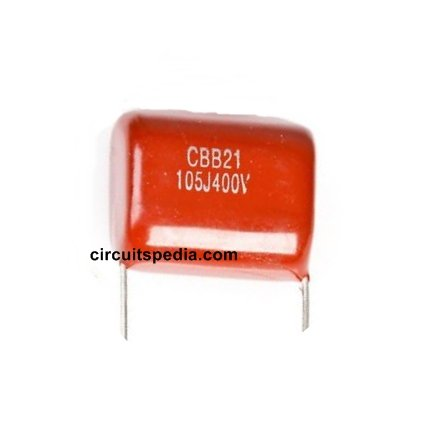105J=10x105 =1000000pf=1000nf=1.0uf

j= +/- 5% Tolerance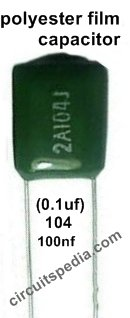104= 10x104= 100000pf=100nf=0.1uf

j=+-5% tolerance

2A= 100VDC voltage ratingHi, My name is Aman bharti, I am interested in making and study of Electronics & circuit diagram. I like to share my knowledge and all ideas with people which i get from My Experiment & from different sources. I try to provide any circuit details deeply with test result as possible as. You can contact me on- Email – [email protected]

5 thoughts on “Very Simple Method to Read Value of Capacitor”

•can u please tell how to identify the values of smd ceramic chip capacitors

•What would the capacitance be for a small through-hole ceramic cap with the code B21 and a horizontal under the code?

•B21 (CBB21) is not capacitor value, this is material specification of capacitor given by manufacturer.
CBB21 denoted Metalized Polypropylene Film capacitor, This is also a type ceramic capacitor

•Very interesting information!Perfect just what I was looking for!

•This is a great tip especially to those new to the blogosphere. Simple but very precise info… Appreciate your sharing this one. A must read post!